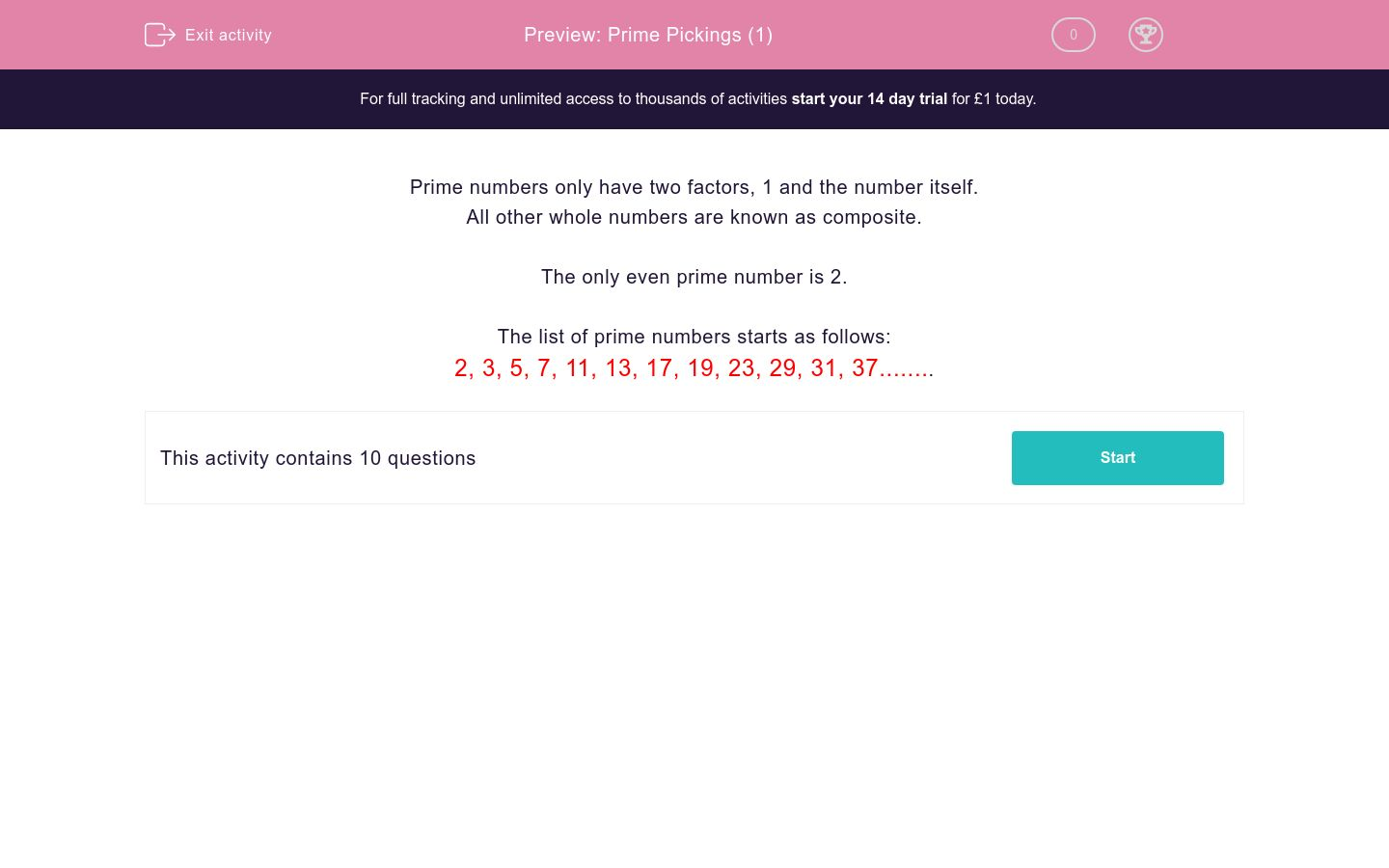# Prime Pickings (1)

In this worksheet, students revise and practise selecting the numbers that are prime.Key stage:  KS 2

Curriculum topic:   Maths and Numerical Reasoning

Curriculum subtopic:   Factors and Multiples

Difficulty level:### QUESTION 1 of 10

Prime numbers only have two factors, 1 and the number itself.

All other whole numbers are known as composite.

The only even prime number is 2.

The list of prime numbers starts as follows:

2, 3, 5, 7, 11, 13, 17, 19, 23, 29, 31, 37........

Select all the numbers which are prime.

2

5

8

9

11

Select all the numbers which are prime.

2

7

13

15

17

Select all the numbers which are prime.

12

15

18

19

23

Select all the numbers which are prime.

17

19

21

23

25

Select all the numbers which are prime.

27

29

31

33

37

Select all the numbers which are prime.

37

39

41

43

45

Select all the numbers which are prime.

49

51

53

57

59

Select all the numbers which are prime.

60

61

67

69

71

Select all the numbers which are prime.

67

71

73

75

79

Select all the numbers which are prime.

37

73

35

53

91

• Question 1

Select all the numbers which are prime.

2
5
11
EDDIE SAYS
8 is not prime as it can be divided by 2 and 4 9 is not prime as it can be divided by 3
• Question 2

Select all the numbers which are prime.

2
7
13
17
EDDIE SAYS
15 is not prime as it can be divided by 3 and 5
• Question 3

Select all the numbers which are prime.

19
23
EDDIE SAYS
12 is not prime as it can be divided by 2,3,4 and 6 15 is not prime as it can be divided by 3 and 5 18 is not prime as it can be divided by 2,3 and 6
• Question 4

Select all the numbers which are prime.

17
19
23
EDDIE SAYS
21 is not prime as it can be divided by 3 and 7 25 is not prime as it can be divided by 5
• Question 5

Select all the numbers which are prime.

29
31
37
EDDIE SAYS
27 is not prime as it can be divided by 3 and 9 33 is not prime as it can be divided by 3 and 11
• Question 6

Select all the numbers which are prime.

37
41
43
EDDIE SAYS
39 is not prime as it can be divided by 3 and 13 45 is not prime as it can be divided by 3, 5, 9 and 15
• Question 7

Select all the numbers which are prime.

53
59
EDDIE SAYS
49 is not prime as it can be divided by 7 51 is not prime as it can be divided by 3 and 17 57 is not prime as it can be divided by 3 and 19
• Question 8

Select all the numbers which are prime.

61
67
71
EDDIE SAYS
60 is not prime as it can be divided by 2, 3, 5, 6, 10, 12, 20 and 30 69 is not prime as it can be divided by 3 and 23
• Question 9

Select all the numbers which are prime.

67
71
73
79
EDDIE SAYS
75 is not prime as it can be divided by 3,5,15 and 25
• Question 10

Select all the numbers which are prime.

37
73
53
EDDIE SAYS
35 is not prime as it can be divided by 5 and 7 91 is not prime as it can be divided by 7 and 13
---- OR ----

Sign up for a £1 trial so you can track and measure your child's progress on this activity.

### What is EdPlace?

We're your National Curriculum aligned online education content provider helping each child succeed in English, maths and science from year 1 to GCSE. With an EdPlace account you’ll be able to track and measure progress, helping each child achieve their best. We build confidence and attainment by personalising each child’s learning at a level that suits them.

Get started